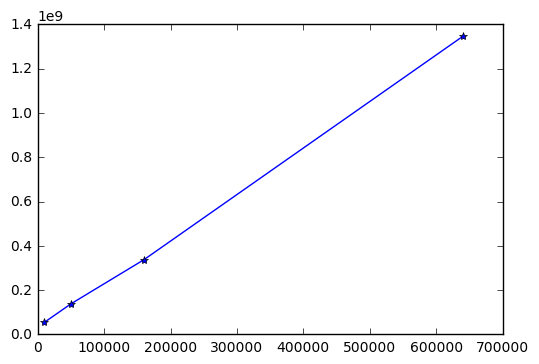# Largest size of image that can be used to train NN

The images in dogscats data set are all under 500px by 500px and the `batch_size` used in the Vgg is 64.

Does this mean using the same GPU, we can increase the resolution of the images to 64 times larger (4000px by 4000px) without running out of memory?

If not, what will be the largest size of images that we can train the cNN with?

The VGG is actually run on 224x224 images – they are resized by the ImageDataGenerator to be 224 x 224. You can size up the images and run on larger images, but you need to remove and retrain the desne layers of VGG (which is covered later in the course if you haven’t reatched that part yet).

If I understand Jeremy right, the batch size is actually important during training – too big doesn’t work well. I’m not sure if going to a batch size of 1 is bad or not. It has to do with how the SGD optimizer works, but I don’t have a full grasp of how that behaves with different batch sizes yet.

So, give it a try with the vgg16bn.py file from github. You can pass size=(400x400) or (4000x4000) parameter to the Vgg16BN() funciton to get your convolutional model. Then you have to add Dense layers on top like is done in the the .py file.

4000x4000 is going to be very big I thinkHere’s what I get for a few different sizes.

I couldn’t create any models larger than about 800 x 800 on the AWS P2 Instances because it ran out of memory (over 61GB) on the host cpu.

``````%matplotlib inline
import matplotlib
import numpy as np
import matplotlib.pyplot as plt

import vgg16bn
from keras.layers.core import Flatten, Dense
import numpy as np

def vgg_weights(size):
vgg = vgg16bn.Vgg16BN(size=size, include_top=False)
vgg.FCBlock()
vgg.FCBlock()
sum = np.sum([np.prod(w.shape) for w in vgg.model.get_weights()])
del vgg
return sum

in_sizes = [ (100, 100), (224, 224), (400, 400), (800,800)]
iss = [x*x for x in in_sizes]
param_sizes = [vgg_weights(size) for size in in_sizes]
``````

### X axis: image size (pixels), y axis (model paramters used)1 Like